# How to Calculate a Five Number Summary in Excel?

• Last Updated : 18 Jul, 2021

In statistics, the five-number summary is mostly used as it gives a rough idea about the dataset. It is basically a summary of the dataset describing some key features in statistics. The five key features are :

1. Minimum value: It is the minimum value in the data set
2. First Quartile, Q1: It is also known as the lower quartile where 25% of the scores fall below it.
3. Median (middle value) or second quartile: It is basically the mid-value in the dataset.
4. Third Quartile, Q3: It is also known as the Upper quartile in which 25% of the data is above it and the rest 75% falls below it.
5. Maximum value: It is the maximum value in the dataset.

It is important to note that we can find the Five-number summary only for a single variable dataset. It is meaningless to calculate the Five-number summary for two variables data also known as bivariate.

These five number summaries give a concise inference about the distribution of the dataset. Using these quartiles we can find the Interquartile Range and create a Box Plot out of it. For more details refer Creating Box Plots in Excel.

In this article, we are going to discuss how to calculate the Five-number summary in Excel using a suitable example.

### Implementation :

The following Excel in-built functions can be used to calculate the above quartiles.

```QUARTILE(Array,quart) // Used in Excel 2007 version and lower

Array : Cell range
quart : The five quart values from 0 to 4

0- Minimum value
1- First Quartile (25 percent)
2- Median Value (50 percentile)
3- Third Quartile (75 percentile)
4- Maximum value```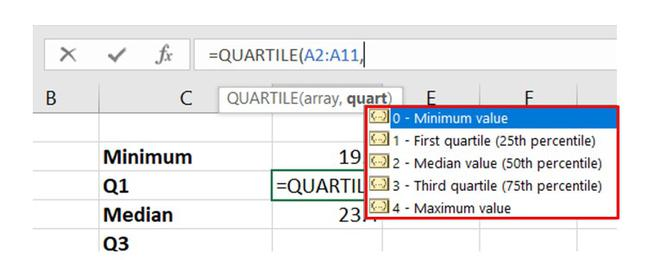In the latest version of Excel, we use the function

`QUARTILE.INC(Array,quart)`

The parameters and quart values are the same as discussed above.

To calculate the minimum, maximum, and median values we can also use the following functions :

```=MIN(Array)        //to calculate minimum value
=MAX(Array)        //to calculate maximum value
=MEDIAN(Array)    //to calculate second quartile or the median```

Example: Consider the dataset which consists of information about the BMI of 10 geeks.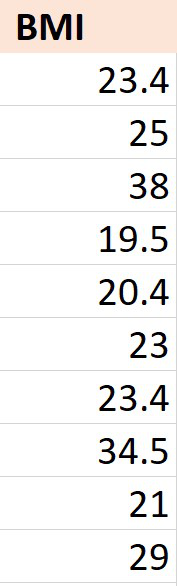Dataset

### CALCULATION:

1. Minimum and Maximum Value

It can either be calculated using the MIN, MAX functions or using the QUARTILE function with quart value as 0 for minimum and 4 for maximum.

The dataset is stored in column “A” of the worksheet and the observations are stored from cell A2 to A11

So the array will start from A2 and end at A11.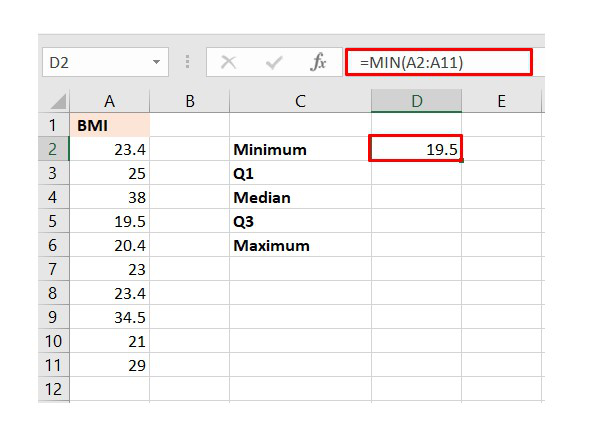Minimum BMI

Similarly, we can find the maximum value.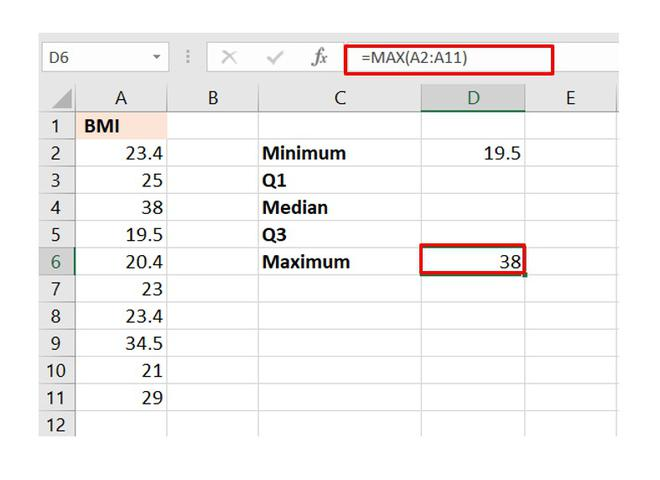Maximum BMI

2. Median value or Second Quartile

The Excel function MEDIAN can be used or we can use the QUARTILE function with quart value as 2.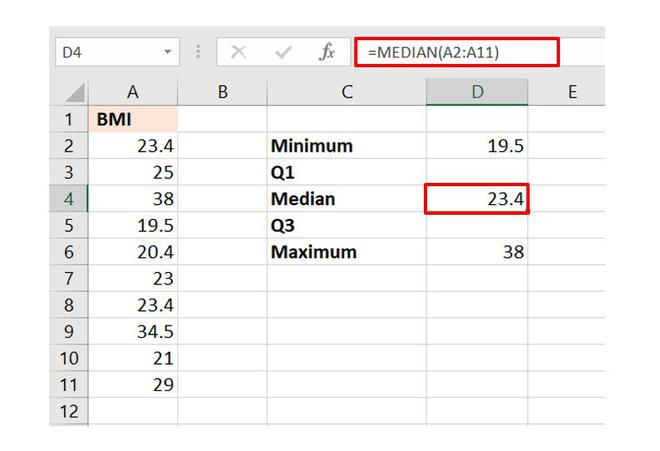3. Quartiles Q1 and Q3

The function QUARTILE or QUARTILE.INC is used to calculate these quartile values with quart values 1 for Q1 and 3 for Q3.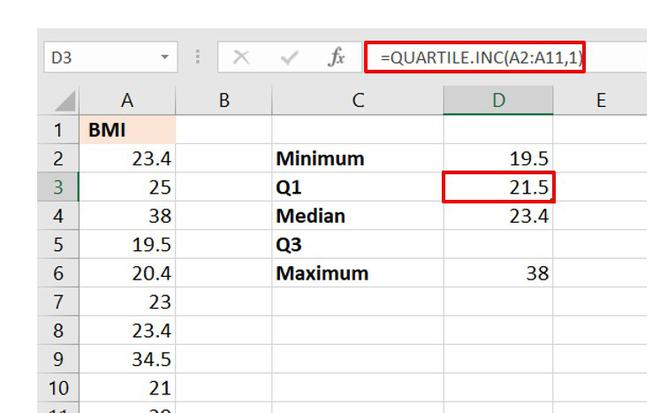Quartile 1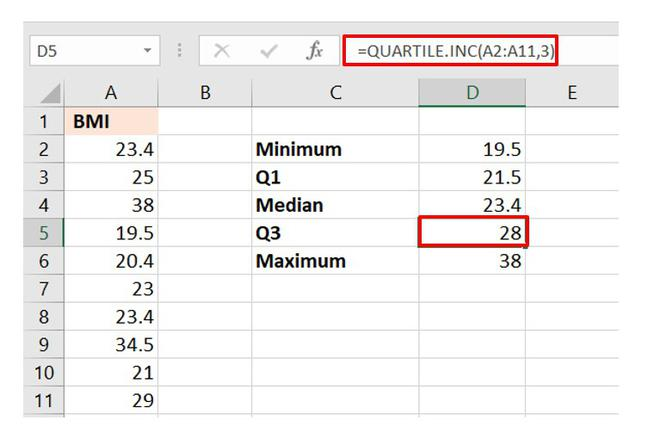Quartile 3

### Summary

To calculate all the Five-number summary :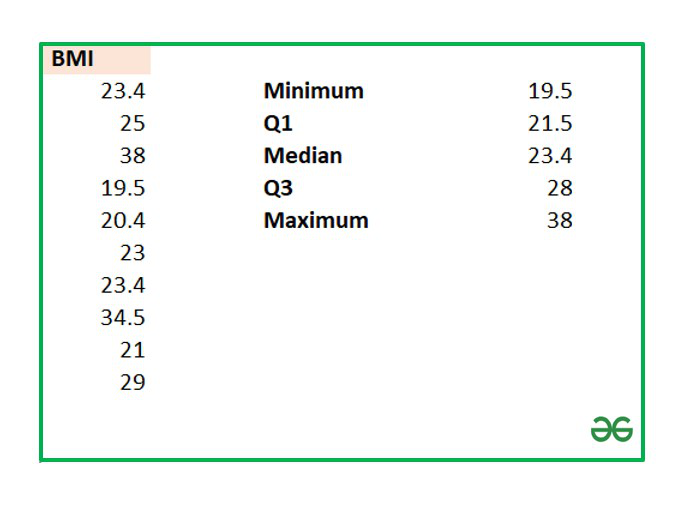Five-Number Summary

My Personal Notes arrow_drop_up